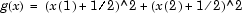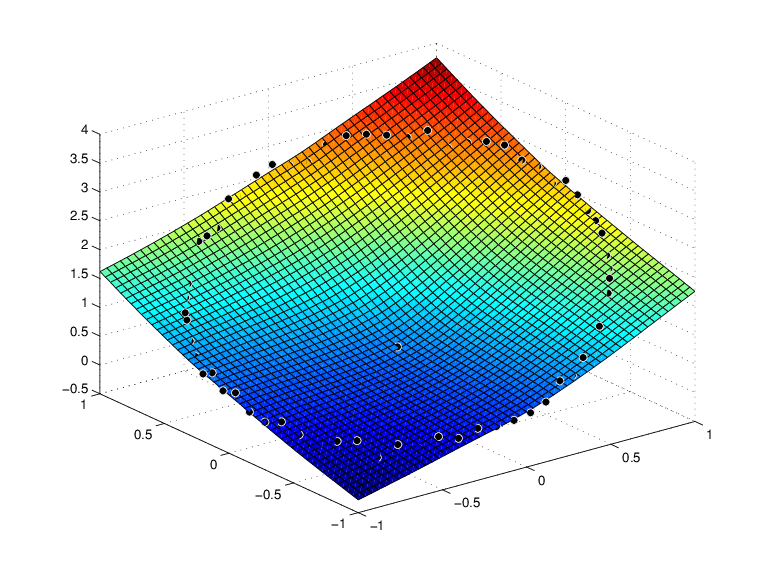## Fitting Values at Scattered 2-D Sites with Thin-Plate Smoothing Splines

Tensor-product splines are good for gridded (bivariate and even multivariate) data. For work with scattered bivariate data, the toolbox provides the thin-plate smoothing spline. Suppose you have given data values `y(j)` at scattered data sites `x(:,j)`, `j=1:N`, in the plane. To give a specific example,

```n = 65; t = linspace(0,2*pi,n+1); x = [cos(t);sin(t)]; x(:,end) = [0;0]; ```

provides 65 sites, namely 64 points equally spaced on the unit circle, plus the center of that circle. Here are corresponding data values, namely noisy values of the very nice function.

```y = (x(1,:)+.5).^2 + (x(2,:)+.5).^2; noisy = y + (rand(size(y))-.5)/3; ```

Then you can compute a reasonable approximation to these data by

```st = tpaps(x,noisy); ```

and plot the resulting approximation along with the noisy data by

```fnplt(st); hold on plot3(x(1,:),x(2,:),noisy,'wo','markerfacecolor','k') hold off ```

and so produce the following picture:

Thin-Plate Smoothing Spline Approximation to Noisy Data